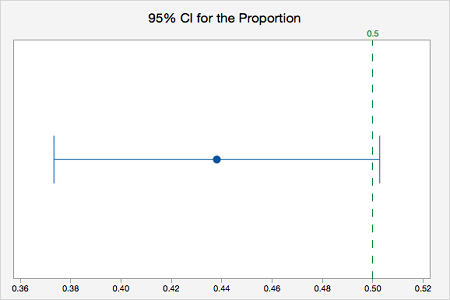# 8.1.2.2.1 - Minitab Express: 1 Proportion z Test, Raw Data

8.1.2.2.1 - Minitab Express: 1 Proportion z Test, Raw Data

If you have data in a Minitab Express worksheet, then you have what we call "raw data."  This is in contrast to "summarized data" which you'll see on the next page.

In order to use the normal approximation method both $np_0 \geq 10$ and $n(1-p_0) \geq 10$. Before we can conduct our hypothesis test we must check this assumption to determine if the normal approximation method or exact method should be used. This must be checked manually. Minitab Express will not check assumptions for you.

In the example below, we want to know if there is evidence that the proportion of students who are male is different from 0.50.

$n=226$ and $p_0=0.50$

$np_0 = 226(0.50)=113$ and $n(1-p_0) = 226(1-0.50)=113$

Both $np_0 \geq 10$ and $n(1-p_0) \geq 10$ so we can use the normal approximation method.

## MinitabExpress – Conducting a One Sample Proportion z Test: Raw Data

Research question: Is the proportion of students who are male different from 0.50?

1. Open Minitab Express file:
2. On a PC: Select STATISTICS > One Sample > Proportion
On a Mac: Select Statistics > 1-Sample Inference > Proportion
3. Double-click the variable Biological Sex to insert it into the Sample box
4. Check the box next to Perform hypothesis test and enter 0.50 in the Hypothesized proportion box
5. Click the Options tab
6. Use the default Alternative hypothesis setting of Proportion ≠ hypothesized value
7. Use the default Confidence level of 95
8. Select Normal approximation method
9. Click OK

The result should be the following output:

Z-Value P-Value
-1.86 0.06251-Sample Proportion: Biological Sex
 Event: Biological Sex = Male p: proportion where Biological Sex = Male Normal approximation is used for this analysis.
Descriptive Statistics
N Event Sample p 95% CI for p
226 99 0.438053 (0.373368, 0.502738)
Null hypothesis H 0: p = 0.5 H 1: p ≠ 0.5
Video Walkthrough

Select your operating system below to see a step-by-step guide for this example.

We could summarize these results using the five-step hypothesis testing procedure:

1. Check assumptions and write hypotheses

$np_0 = 226(0.50)=113$ and $n(1-p_0) = 226(1-0.50)=113$ therefore the normal approximation method will be used.

$H_0: p = 0.50$

$H_a: p \ne 0.50$

2. Calculate the test statistic

From the Minitab Express output, $z$ = -1.86

3. Determine the p-value

From the Minitab Express output, $p$ = 0.0625

4. Make a decision

$p > \alpha$, fail to reject the null hypothesis

5. State a "real world" conclusion

There is NOT evidence that the proportion of all students in the population who are male is different from 0.50.

  Link ↥ Has Tooltip/Popover Toggleable Visibility